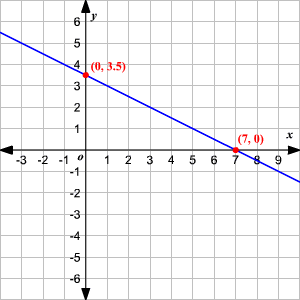# $x$ -Intercepts

The $x$ -intercepts of a function are the point(s) where the graph of the function crosses the $x$ -axis.

The $x$ -intercept is often referred to with just the $x$ -value. For example, we say that the $x$ -intercept of the line shown in the graph below is $7$ .